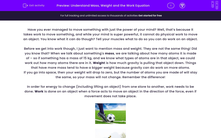# Understand Mass, Weight and the Work Equation

In this worksheet, students will learn the difference between mass and weight, as well as using the work done equation.Key stage:  KS 4

Year:  GCSE

GCSE Boards:   AQA, AQA Trilogy, AQA Synergy, OCR 21st Century, OCR Gateway, Pearson Edexcel, Eduqas,

Popular topics:   Physics worksheets

Difficulty level:#### Worksheet Overview

Have you ever managed to move something with just the power of your mind? Well, that’s because it takes work to move something, and while your mind is super powerful, it cannot do physical work to move an object. You know what it can do though? Tell your muscles what to do so you can do work on an object.

Before we get into work though, I just want to mention mass and weight. They are not the same thing! Did you know that? When we talk about something's mass, we are talking about how many atoms it is made of – so if something has a mass of 15 kg, and we know what types of atoms are in that object, we could work out how many atoms there are in it. Weight is how much gravity is pulling that object down. Things that have more mass tend to have a bigger weight because gravity can do work on more atoms.

If you go into space, then your weight will drop to zero, but the number of atoms you are made of will stay the same, so your mass will not change. Remember the difference!

In order for energy to change (including lifting an object) from one store to another, work needs to be done. Work is done on an object when a force acts to move an object in the direction of the force, even if movement does not take place.Work is essentially the amount of energy that has changed form. This can be calculated using the following formula:

work  =  force  ×  distance travelled

The force is measured in Newtons (N) and the distance in metres (m). The unit for work is joules (J).

This equation tells us that if you increase the force or the distance an object travels, then you will increase the work as well.

Let's have a go at an example:

Sarah moves an object that weighs 500 N a distance of 3 m. How much work has she done?

1   Highlight all of the numbers:

Sarah moves an object that weighs 500 N a distance of 3 m. How much work has she done?

2   Write down what they mean:

Weight (force) = 500 N

Distance = 3 m

3   Put them into the equation:

Work done = 500 x 3

4   Do the sum on your calculator and write down the answer:

Work done = 15000 J.

Job done! Let's have a go at some questions now to test out this equation.

### What is EdPlace?

We're your National Curriculum aligned online education content provider helping each child succeed in English, maths and science from year 1 to GCSE. With an EdPlace account you’ll be able to track and measure progress, helping each child achieve their best. We build confidence and attainment by personalising each child’s learning at a level that suits them.

Get started